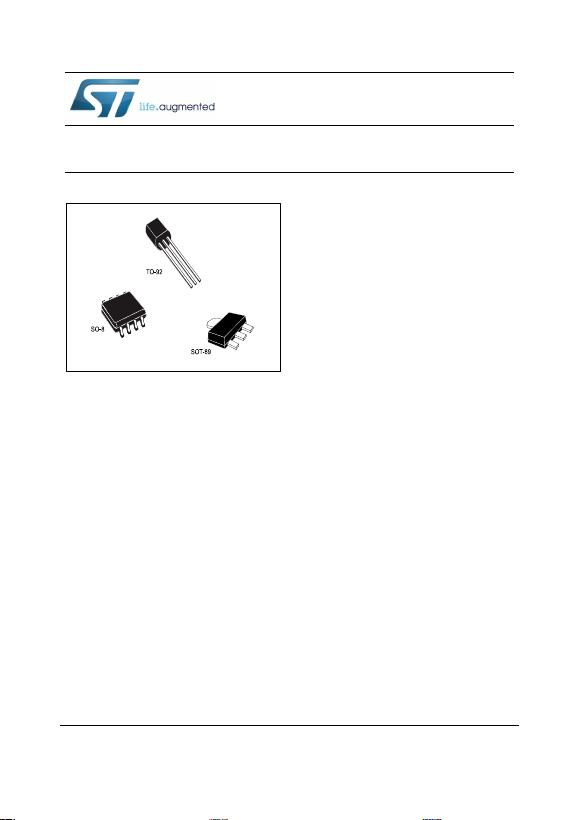June 2016
DocID2145 Rev 26
1/45
This is information on a product in full production.
www.st.com
L78L
Positive voltage regulators
Datasheet - production data
Features
Output current up to 100 mA
Output voltages of 3.3; 5; 6; 8; 9; 10; 12; 15;
18; 24 V thermal overload protection
Short-circuit protection
No external components are required
Available in either ± 4% (A) or ± 8% (C)
selection
Description
The L78L series of three-terminal positive
regulators employ internal current limiting and
thermal shutdown, making them essentially
indestructible. If adequate heat-sink is provided,
they can deliver up to 100 mA output current.
They are intended as fixed voltage regulators in a
wide range of applications including local or on-
card regulation for elimination of noise and
distribution problems associated with single-point
regulation. In addition, they can be used with
power pass elements to make high-current
voltage regulators. The L78L series used as
Zener diode/resistor combination replacement,
offers e improvement along with lower quiescent
current and lower noise.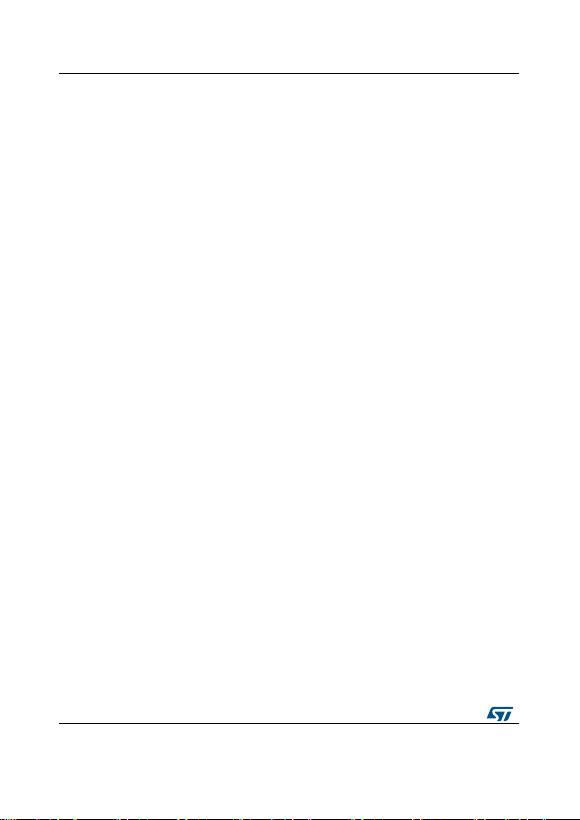Contents
2/45
DocID2145 Rev 26
Contents
1 Diagram ............................................................................................ 3
2 Pin configuration ............................................................................. 4
3 Maximum ratings ............................................................................. 5
4 Electrical characteristics ................................................................ 6
5 Typical performance ..................................................................... 25
6 Typical application ........................................................................ 27
7 Package information ..................................................................... 29
7.1 TO-92 package information ............................................................. 29
7.2 TO-92 packing information .............................................................. 30
7.3 TO-92 Ammopak packing information ............................................. 32
7.4 SO-8 package information .............................................................. 34
7.5 SO-8 packing information ................................................................ 36
7.6 SOT-89 package information .......................................................... 37
7.7 SOT-89 packing information............................................................ 40
8 Ordering information ..................................................................... 41
9 Revision history ............................................................................ 44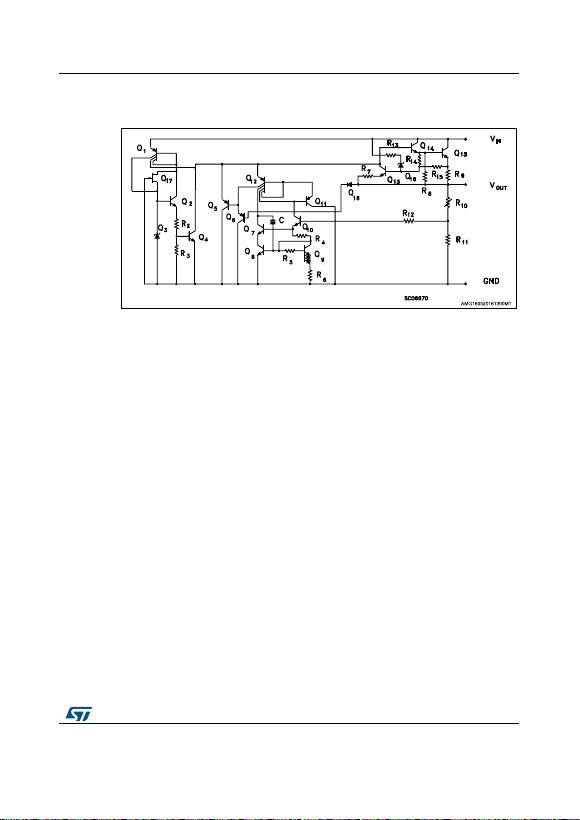L78L
Diagram
DocID2145 Rev 26
3/45
1 Diagram
Figure 1: Schematic diagram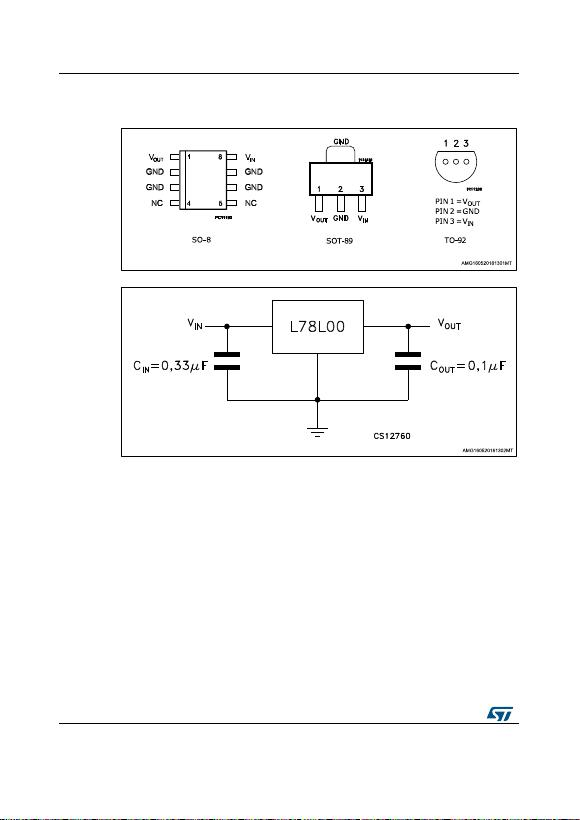Pin configuration
L78L
4/45
DocID2145 Rev 26
2 Pin configuration
Figure 2: Pin connection (top view, bottom view for TO-92)
Figure 3: Test circuits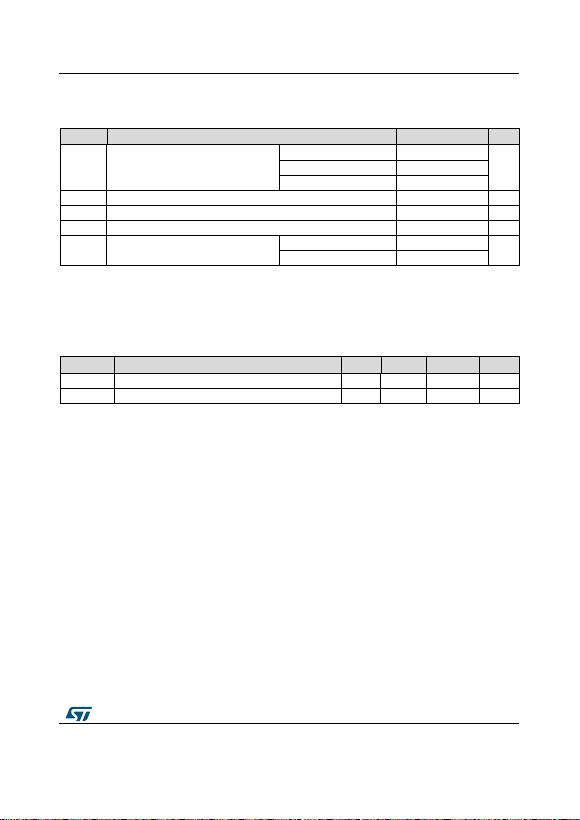L78L
Maximum ratings
DocID2145 Rev 26
5/45
3 Maximum ratings
Table 1: Absolute maximum ratings
Symbol
Parameter
Value
Unit
VI
DC Input voltage
VO = 3.3 to 9 V
30
V
VO = 12 to 15 V
35
VO = 18 to 24 V
40
IO
Output current
100
mA
PD
Power dissipation
Internally limited (1)
mW
TSTG
Storage temperature range
-65 to 150
°C
TOP
Operating junction temperature range
for L78LxxAC / L78LxxC
0 to 125
°C
for L78LxxAB
-40 to 125
Notes:
(1)Our SO-8 package used for voltage regulators is modified internally to have pins 2, 3, 6 and 7 electrically communed to the die
attach flag. This particular frame decreases the total thermal resistance of the package and increases its ability to dissipate power
when an appropriate area of copper on the printed circuit board is available for heat-sinking. The external dimensions are the
same as for the standard SO-8.
Table 2: Thermal data
Symbol
Parameter
SO-8
TO-92
SOT-89
Unit
RthJC
Thermal resistance junction-case (max)
20
15
°C/W
RthJA
Thermal resistance junction-ambient (max)
55 (1)
200
55 (1)
°C/W
Notes:
(1)Considering 6 cm² of copper Board heat-sink.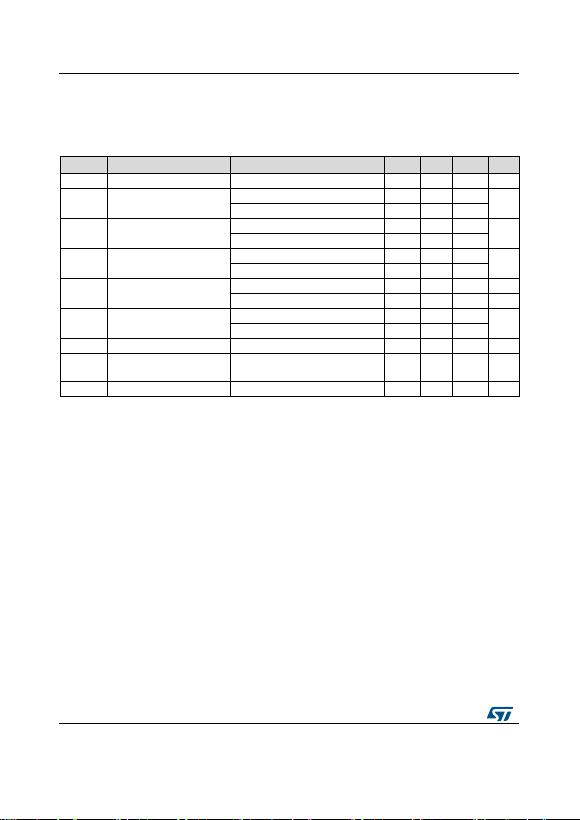Electrical characteristics
L78L
6/45
DocID2145 Rev 26
4 Electrical characteristics
Refer to the test circuits, TJ = 0 to 125 °C, VI = 8.3 V, IO = 40 mA, CI = 0.33 µF, CO = 0.1 µF
unless otherwise specified.
Table 3: Electrical characteristics of L78L33C
Symbol
Parameter
Test conditions
Min.
Typ.
Max.
Unit
VO
Output voltage
TJ = 25 °C
3.036
3.3
3.564
V
VO
Output voltage
IO = 1 to 40 mA, VI = 5.3 to 20 V
2.97
3.63
V
IO = 1 to 70 mA, VI = 8.3 V
2.97
3.63
∆VO
Line regulation
VI = 5.4 to 20 V, TJ = 25 °C
150
mV
VI = 6.3 to 20 V, TJ = 25 °C
100
∆VO
IO = 1 to 100 mA, TJ = 25 °C
60
mV
IO = 1 to 40 mA, TJ = 25 °C
30
Id
Quiescent current
TJ = 25 °C
6
mA
TJ = 125 °C
5.5
mA
∆Id
Quiescent current change
IO = 1 to 40 mA
0.2
mA
VI = 6.3 to 20 V
1.5
eN
Output noise voltage
B = 10 Hz to 100 kHz, TJ = 25 °C
40
µV
SVR
Supply voltage rejection
VI = 6.3 to 16.3 V, f = 120 Hz
IO = 40 mA, TJ = 25 °C
41
49
dB
Vd
Dropout voltage
2
V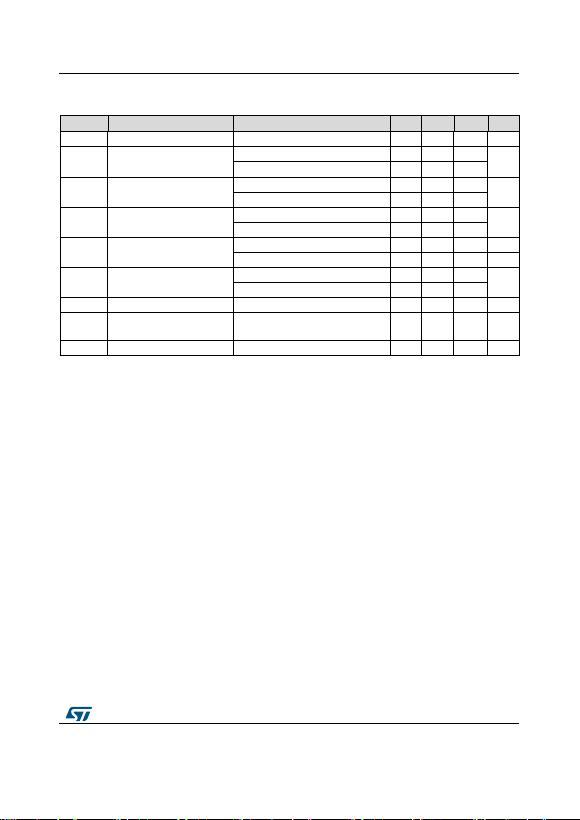L78L
Electrical characteristics
DocID2145 Rev 26
7/45
Refer to the test circuits, TJ = 0 to 125 °C, VI = 10 V, IO = 40 mA, CI = 0.33 µF, CO = 0.1 µF
unless otherwise specified.
Table 4: Electrical characteristics of L78L05C
Symbol
Parameter
Test conditions
Min.
Typ.
Max.
Unit
VO
Output voltage
TJ = 25 °C
4.6
5
5.4
V
VO
Output voltage
IO = 1 to 40 mA, VI = 7 to 20 V
4.5
5.5
V
IO = 1 to 70 mA, VI = 10 V
4.5
5.5
∆VO
Line regulation
VI = 8.5 to 20 V, TJ = 25 °C
200
mV
VI = 9 to 20 V, TJ = 25 °C
150
∆VO
IO = 1 to 100 mA, TJ = 25 °C
60
mV
IO = 1 to 40 mA, TJ = 25 °C
30
Id
Quiescent current
TJ = 25 °C
6
mA
TJ = 125 °C
5.5
mA
Id
Quiescent current change
IO = 1 to 40 mA
0.2
mA
VI = 8 to 20 V
1.5
eN
Output noise voltage
B = 10 Hz to 100 kHz, TJ = 25 °C
40
µV
SVR
Supply voltage rejection
VI = 9 to 20 V, f = 120 Hz
IO = 40 mA, TJ = 25 °C
40
49
dB
Vd
Dropout voltage
2
V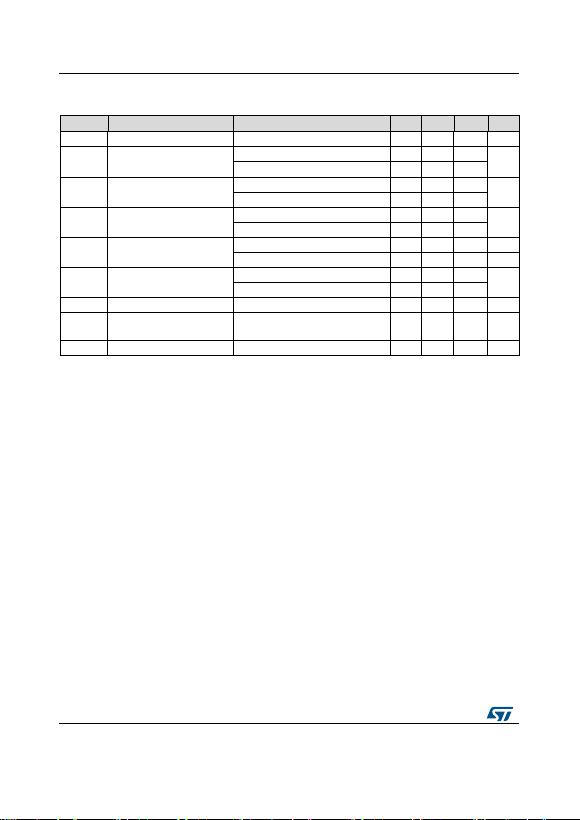Electrical characteristics
L78L
8/45
DocID2145 Rev 26
Refer to the test circuits, TJ = 0 to 125 °C,VI = 14 V, IO = 40 mA, CI = 0.33 µF, CO = 0.1 µF
unless otherwise specified.
Table 5: Electrical characteristics of L78L08C
Symbol
Parameter
Test conditions
Min.
Typ.
Max.
Unit
VO
Output voltage
TJ = 25 °C
7.36
8
8.64
V
VO
Output voltage
IO = 1 to 40 mA, VI = 8.5 to 20 V
7.2
8.8
V
IO = 1 to 70 mA, VI = 12 V
7.2
8.8
∆VO
Line regulation
VI = 10.5 to 20 V, TJ = 25 °C
200
mV
VI = 11 to 20 V, TJ = 25 °C
150
∆VO
IO = 1 to 100 mA, TJ = 25 °C
80
mV
IO = 1 to 40 mA, TJ = 25 °C
40
Id
Quiescent current
TJ = 25 °C
6
mA
TJ = 125 °C
5.5
mA
∆Id
Quiescent current change
IO = 1 to 40 mA
0.2
mA
VI = 8 to 20 V
1.5
eN
Output noise voltage
B = 10 Hz to 100 kHz, TJ = 25 °C
60
µV
SVR
Supply voltage rejection
VI = 9 to 20 V, f = 120 Hz
IO = 40 mA, TJ = 25 °C
36
45
dB
Vd
Dropout voltage
1.7
V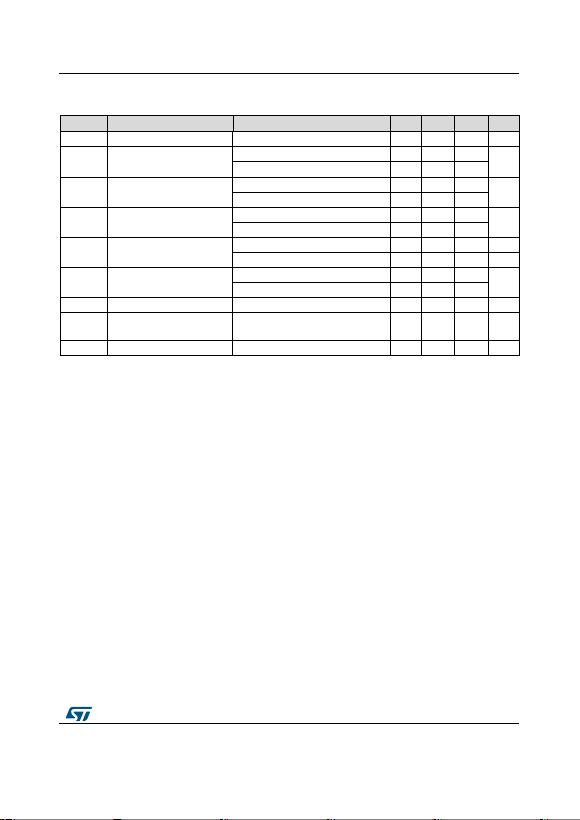L78L
Electrical characteristics
DocID2145 Rev 26
9/45
Refer to the test circuits, TJ = 0 to 125 °C, VI = 15 V, IO = 40 mA, CI = 0.33 µF, CO = 0.1 µF
unless otherwise specified.
Table 6: Electrical characteristics of L78L09C
Symbol
Parameter
Test conditions
Min.
Typ.
Max.
Unit
VO
Output voltage
TJ = 25 °C
8.28
9
9.72
V
VO
Output voltage
IO = 1 to 40 mA, VI = 11.5 to 23 V
8.1
9.9
V
IO = 1 to 70 mA, VI = 15 V
8.1
9.9
∆VO
Line regulation
VI = 11.5 to 23 V, TJ = 25 °C
250
mV
VI = 12 to 23 V, TJ = 25 °C
200
∆VO
IO = 1 to 100 mA, TJ = 25 °C
80
mV
IO = 1 to 40 mA, TJ = 25 °C
40
Id
Quiescent current
TJ = 25 °C
6
mA
TJ = 125 °C
5.5
mA
∆Id
Quiescent current change
IO = 1 to 40 mA
0.2
mA
VI = 12 to 23 V
1.5
eN
Output noise voltage
B = 10 Hz to 100 kHz, TJ = 25 °C
70
µV
SVR
Supply voltage rejection
VI = 12 to 23 V, f = 120 Hz
IO = 40 mA, TJ = 25 °C
36
44
dB
Vd
Dropout voltage
1.7
V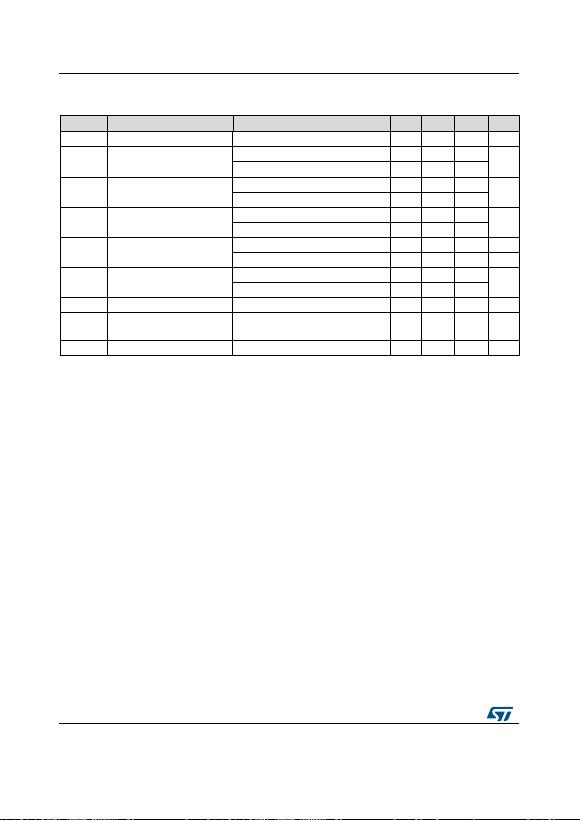Electrical characteristics
L78L
10/45
DocID2145 Rev 26
Refer to the test circuits, TJ = 0 to 125 °C, VI = 16 V, IO = 40 mA, CI = 0.33 µF, CO = 0.1 µF
unless otherwise specified.
Table 7: Electrical characteristics of L78L10C
Symbol
Parameter
Test conditions
Min.
Typ.
Max.
Unit
VO
Output voltage
TJ = 25 °C
9.2
10
10.8
V
VO
Output voltage
IO = 1 to 40 mA, VI = 12.5 to 23 V
9
11
V
IO = 1 to 70 mA, VI = 16 V
9
11
∆VO
Line regulation
VI = 12.5 to 23 V, TJ = 25 °C
230
mV
VI = 13 to 23 V, TJ = 25 °C
170
∆VO
IO = 1 to 100 mA, TJ = 25 °C
80
mV
IO = 1 to 40 mA, TJ = 25 °C
40
Id
Quiescent current
TJ = 25 °C
6
mA
TJ = 125 °C
5.5
mA
∆Id
Quiescent current change
IO = 1 to 40 mA
0.1
mA
VI = 13 to 23 V
1.5
eN
Output noise voltage
B = 10 Hz to 100 kHz, TJ = 25 °C
60
µV
SVR
Supply voltage rejection
VI = 14 to 23 V, f = 120 Hz
IO = 40 mA, TJ = 25 °C
37
45
dB
Vd
Dropout voltage
1.7
V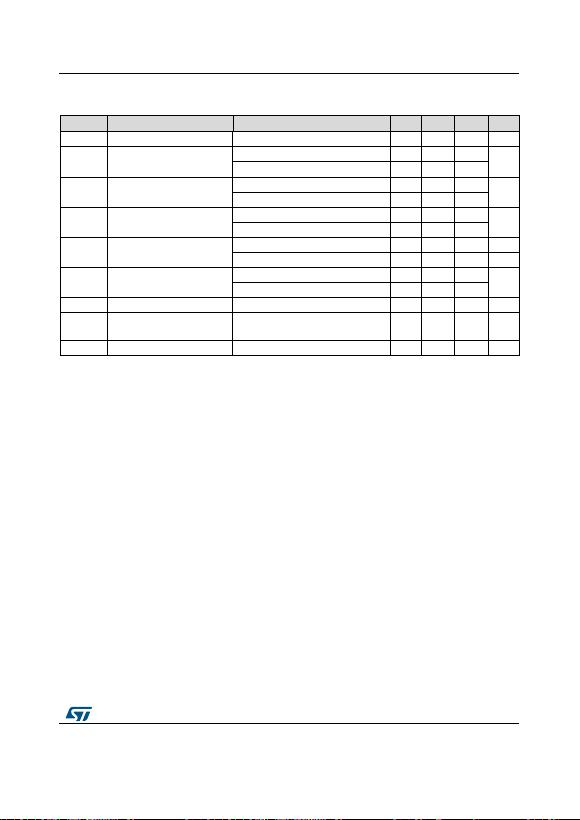L78L
Electrical characteristics
DocID2145 Rev 26
11/45
Refer to the test circuits, TJ = 0 to 125 °C, VI = 19 V, IO = 40 mA, CI = 0.33 µF, CO = 0.1 µF
unless otherwise specified.
Table 8: Electrical characteristics of L78L12C
Symbol
Parameter
Test conditions
Min.
Typ.
Max.
Unit
VO
Output voltage
TJ = 25 °C
11.1
12
12.9
V
VO
Output voltage
IO = 1 to 40 mA, VI = 14.5 to 27 V
10.8
13.2
V
IO = 1 to 70 mA, VI = 19 V
10.8
13.2
∆VO
Line regulation
VI = 14.5 to 27 V, TJ = 25 °C
250
mV
VI = 16 to 27 V, TJ = 25 °C
200
∆VO
IO = 1 to 100 mA, TJ = 25 °C
100
mV
IO = 1 to 40 mA, TJ = 25 °C
50
Id
Quiescent current
TJ = 25 °C
6.5
mA
TJ = 125 °C
6
mA
∆Id
Quiescent current change
IO = 1 to 40 mA
0.2
mA
VI = 16 to 27 V
1.5
eN
Output noise voltage
B = 10 Hz to 100 kHz, TJ = 25 °C
80
µV
SVR
Supply voltage rejection
VI = 15 to 25 V, f = 120 Hz
IO = 40 mA, TJ = 25 °C
36
42
dB
Vd
Dropout voltage
1.7
V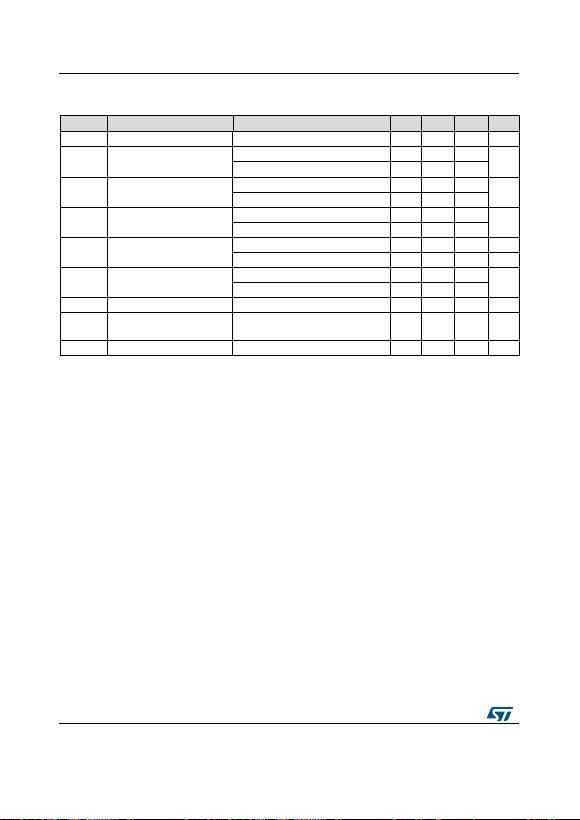Electrical characteristics
L78L
12/45
DocID2145 Rev 26
Refer to the test circuits, TJ = 0 to 125 °C, VI = 23 V, IO = 40 mA, CI = 0.33 µF, CO = 0.1 µF
unless otherwise specified
Table 9: Electrical characteristics of L78L15C
Symbol
Parameter
Test conditions
Min.
Typ.
Max.
Unit
VO
Output voltage
TJ = 25 °C
13.8
15
16.2
V
VO
Output voltage
IO = 1 to 40 mA, VI = 17.5 to 30 V
13.5
16.5
V
IO = 1 to 70 mA, VI = 23 V
13.5
16.5
∆VO
Line regulation
VI = 17.5 to 30 V, TJ = 25 °C
300
mV
VI = 20 to 30 V, TJ = 25 °C
250
∆VO
IO = 1 to 100 mA, TJ = 25 °C
150
mV
IO = 1 to 40 mA, TJ = 25 °C
75
Id
Quiescent current
TJ = 25 °C
6.5
mA
TJ = 125 °C
6
mA
∆Id
Quiescent current change
IO = 1 to 40 mA
0.2
mA
VI = 20 to 30 V
1.5
eN
Output noise voltage
B = 10 Hz to 100 kHz, TJ = 25 °C
90
µV
SVR
Supply voltage rejection
VI = 18.5 to 28.5 V, f = 120 Hz
IO = 40 mA, TJ = 25 °C
33
39
dB
Vd
Dropout voltage
1.7
V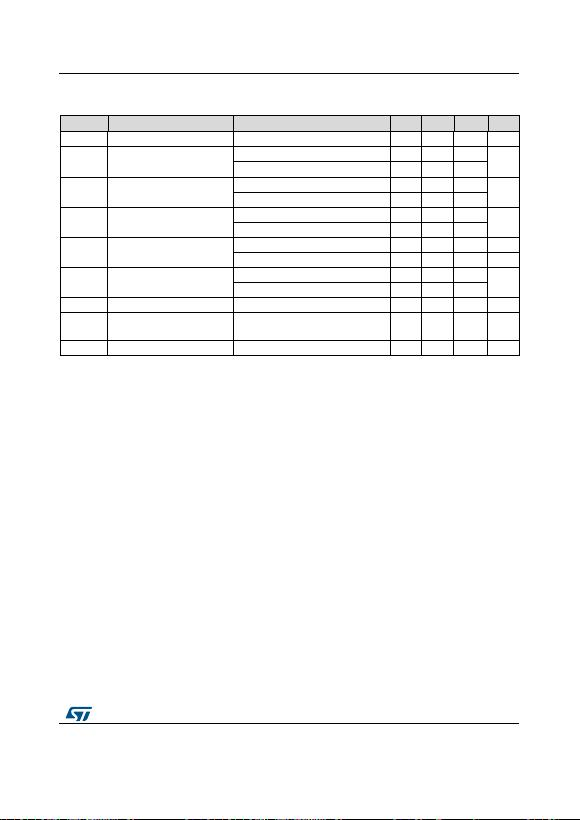L78L
Electrical characteristics
DocID2145 Rev 26
13/45
Refer to the test circuits, TJ = 0 to 125 °C, VI = 27 V, IO = 40 mA, CI = 0.33 µF, CO = 0.1 µF
unless otherwise specified.
Table 10: Electrical characteristics of L78L18C
Symbol
Parameter
Test conditions
Min.
Typ.
Max.
Unit
VO
Output voltage
TJ = 25 °C
16.6
18
19.4
V
VO
Output voltage
IO = 1 to 40 mA, VI = 22 to 33 V
16.2
19.8
V
IO = 1 to 70 mA, VI = 27 V
16.2
19.8
∆VO
Line regulation
VI = 22 to 33 V, TJ = 25 °C
320
mV
VI = 22 to 33 V, TJ = 25 °C
270
∆VO
IO = 1 to 100 mA, TJ = 25 °C
170
mV
IO = 1 to 40 mA, TJ = 25 °C
85
Id
Quiescent current
TJ = 25 °C
6.5
mA
TJ = 125 °C
6
mA
∆Id
Quiescent current change
IO = 1 to 40 mA
0.2
mA
VI = 23 to 33 V
1.5
eN
Output noise voltage
B = 10 Hz to 100 kHz, TJ = 25 °C
120
µV
SVR
Supply voltage rejection
VI = 23 to 33 V, f = 120 Hz
IO = 40 mA, TJ = 25 °C
32
38
dB
Vd
Dropout voltage
1.7
V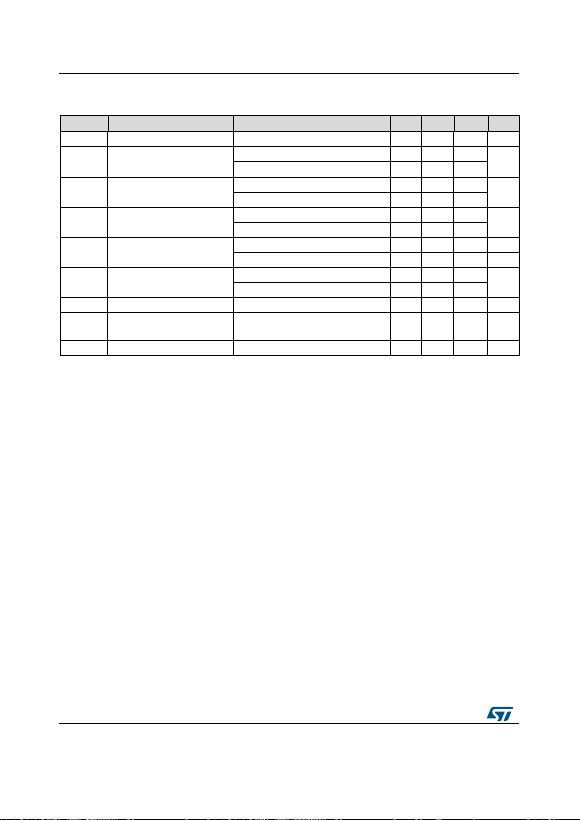Electrical characteristics
L78L
14/45
DocID2145 Rev 26
Refer to the test circuits, TJ = 0 to 125 °C, VI = 33 V, IO = 40 mA, CI = 0.33 µF, CO = 0.1 µF
unless otherwise specified.
Table 11: Electrical characteristics of L78L24C
Symbol
Parameter
Test conditions
Min.
Typ.
Max.
Unit
VO
Output voltage
TJ = 25 °C
22.1
24
25.9
V
VO
Output voltage
IO = 1 to 40 mA, VI = 27 to 38 V
21.6
26.4
V
IO = 1 to 70 mA, VI = 33 V
21.6
26.4
∆VO
Line regulation
VI = 27 to 38 V, TJ = 25 °C
350
mV
VI = 28 to 38 V, TJ = 25 °C
300
∆VO
IO = 1 to 100 mA, TJ = 25 °C
200
mV
IO = 1 to 40 mA, TJ = 25 °C
100
Id
Quiescent current
TJ = 25 °C
6.5
mA
TJ = 125 °C
6
mA
∆Id
Quiescent current change
IO = 1 to 40 mA
0.2
mA
VI = 28 to 38 V
1.5
eN
Output noise voltage
B = 10 Hz to 100 kHz, TJ = 25 °C
200
µV
SVR
Supply voltage rejection
VI = 29 to 35 V, f = 120 Hz
IO = 40 mA, TJ = 25 °C
30
37
dB
Vd
Dropout voltage
1.7
V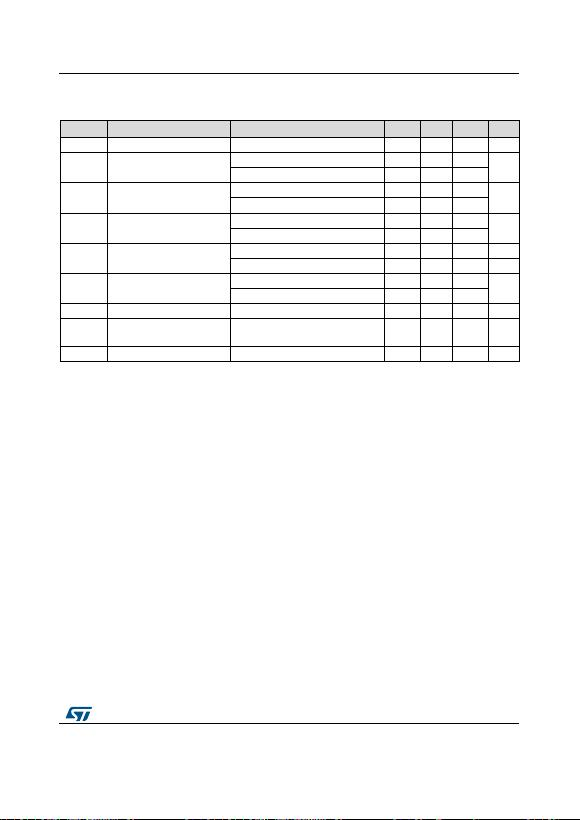L78L
Electrical characteristics
DocID2145 Rev 26
15/45
Refer to the test circuits, TJ = 0 to 125 °C (AC) TJ = -40 to 125 °C (AB),VI = 8.3 V,
IO = 40mA, CI = 0.33 μF, CO = 0.1 μF unless otherwise specified.
Table 12: Electrical characteristics of L78L33AB and L78L33AC
Symbol
Parameter
Test conditions
Min.
Typ.
Max.
Unit
VO
Output voltage
TJ = 25 °C
3.168
3.3
3.432
V
VO
Output voltage
IO = 1 to 40 mA, VI = 5.3 to 20 V
3.135
3.465
V
IO = 1 to 70 mA, VI = 8.3 V
3.135
3.465
∆VO
Line regulation
VI = 5.4 to 20 V, TJ = 25 °C
150
mV
VI = 6.3 to 20 V, TJ = 25 °C
100
∆VO
IO = 1 to 100 mA, TJ = 25 °C
60
mV
IO = 1 to 40 mA, TJ = 25 °C
30
Id
Quiescent current
TJ = 25 °C
6
mA
TJ = 125 °C
5.5
mA
∆Id
Quiescent current change
IO = 1 to 40 mA
0.1
mA
VI = 6.3 to 20 V
1.5
eN
Output noise voltage
B = 10 Hz to 100 kHz, TJ = 25 °C
40
µV
SVR
Supply voltage rejection
VI = 6.3 to 16.3 V, f = 120 Hz
IO = 40 mA, TJ = 25 °C
41
49
dB
Vd
Dropout voltage
2
V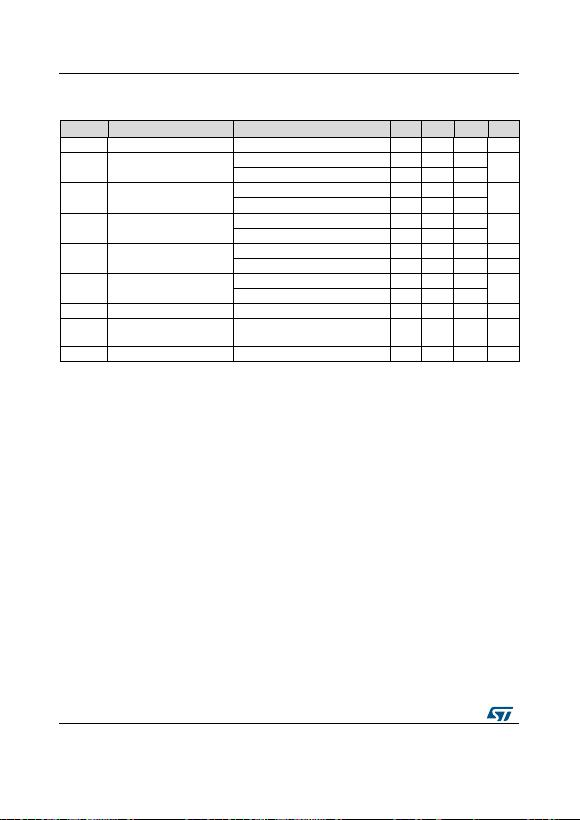Electrical characteristics
L78L
16/45
DocID2145 Rev 26
Refer to the test circuits, TJ = 0 to 125 °C (AC) TJ = -40 to 125 °C (AB), VI = 10 V,
IO = 40 mA, CI = 0.33 μF, CO = 0.1 μF unless otherwise specified.
Table 13: Electrical characteristics of L78L05AB and L78L05AC
Symbol
Parameter
Test conditions
Min.
Typ.
Max.
Unit
VO
Output voltage
TJ = 25 °C
4.8
5
5.2
V
VO
Output voltage
IO = 1 to 40 mA, VI = 7 to 20 V
4.75
5.25
V
IO = 1 to 70 mA, VI = 10 V
4.75
5.25
∆VO
Line regulation
VI = 7.3 to 20 V, TJ = 25 °C
150
mV
VI = 8 to 20 V, TJ = 25 °C
100
∆VO
IO = 1 to 100 mA, TJ = 25 °C
60
mV
IO = 1 to 40 mA, TJ = 25 °C
30
Id
Quiescent current
TJ = 25 °C
6
mA
TJ = 125 °C
5.5
mA
∆Id
Quiescent current change
IO = 1 to 40 mA
0.1
mA
VI = 8 to 20 V
1.5
eN
Output noise voltage
B = 10 Hz to 100 kHz, TJ = 25 °C
40
µV
SVR
Supply voltage rejection
VI = 8 to 18 V, f = 120 Hz
IO = 40 mA, TJ = 25 °C
41
49
dB
Vd
Dropout voltage
2
V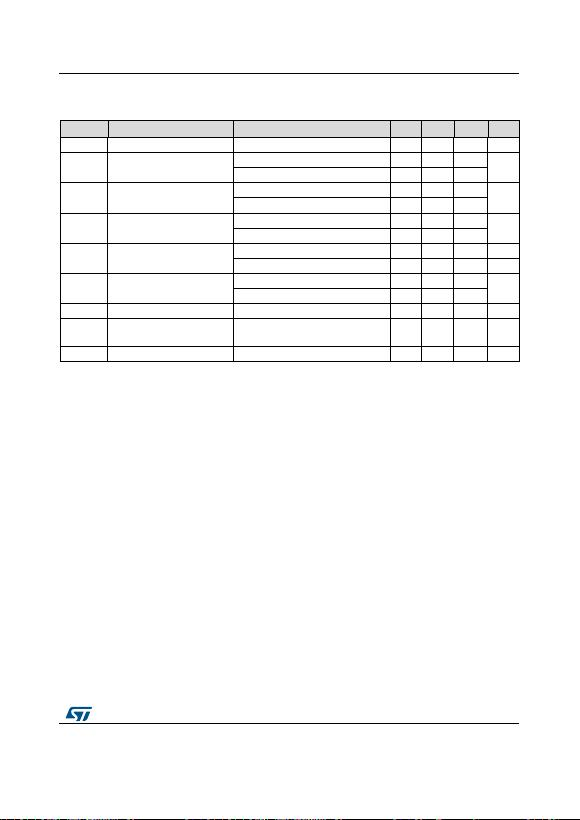L78L
Electrical characteristics
DocID2145 Rev 26
17/45
Refer to the test circuits, TJ = 0 to 125 °C (AC) TJ = -40 to 125 °C (AB), VI = 12 V,
IO = 40 mA, CI = 0.33 μF, CO = 0.1 μF unless otherwise specified.
Table 14: Electrical characteristics of L78L06AB and L78L06AC
Symbol
Parameter
Test conditions
Min.
Typ.
Max.
Unit
VO
Output voltage
TJ = 25 °C
5.76
6
6.24
V
VO
Output voltage
IO = 1 to 40 mA, VI = 8.5 to 20 V
5.7
6.3
V
IO = 1 to 70 mA, VI = 12 V
5.7
6.3
∆VO
Line regulation
VI = 8.5 to 20 V, TJ = 25 °C
150
mV
VI = 9 to 20 V, TJ = 25 °C
100
∆VO
IO = 1 to 100 mA, TJ = 25 °C
60
mV
IO = 1 to 40 mA, TJ = 25 °C
30
Id
Quiescent current
TJ = 25 °C
6
mA
TJ = 125 °C
5.5
mA
∆Id
Quiescent current change
IO = 1 to 40 mA
0.1
mA
VI = 9 to 20 V
1.5
eN
Output noise voltage
B = 10 Hz to 100 kHz, TJ = 25 °C
50
µV
SVR
Supply voltage rejection
VI = 9 to 20 V, f = 120 Hz
IO = 40 mA, TJ = 25 °C
39
46
dB
Vd
Dropout voltage
1.7
V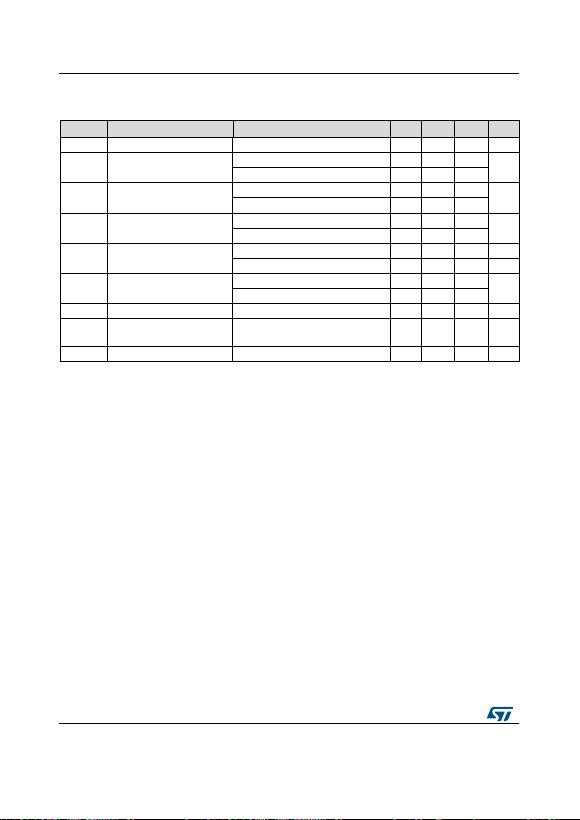Electrical characteristics
L78L
18/45
DocID2145 Rev 26
Refer to the test circuits, TJ = 0 to 125 °C (AC) TJ = -40 to 125 °C (AB), VI = 14 V,
IO = 40 mA, CI = 0.33 μF, CO = 0.1 μF unless otherwise specified.
Table 15: Electrical characteristics of L78L08AB and L78L08AC
Symbol
Parameter
Test conditions
Min.
Typ.
Max.
Unit
VO
Output voltage
TJ = 25 °C
7.68
8
8.32
V
VO
Output voltage
IO = 1 to 40 mA, VI = 10.5 to 23 V
7.6
8.4
V
IO = 1 to 70 mA, VI = 14 V
7.6
8.4
∆VO
Line regulation
VI = 10.5 to 23 V, TJ = 25 °C
175
mV
VI = 11 to 23 V, TJ = 25 °C
125
∆VO
IO = 1 to 100 mA, TJ = 25 °C
80
mV
IO = 1 to 40 mA, TJ = 25 °C
40
Id
Quiescent current
TJ = 25 °C
6
mA
TJ = 125 °C
5.5
mA
∆Id
Quiescent current change
IO = 1 to 40 mA
0.1
mA
VI = 11 to 23 V
1.5
eN
Output noise voltage
B = 10 Hz to 100 kHz, TJ = 25 °C
60
µV
SVR
Supply voltage rejection
VI = 12 to 23 V, f = 120 Hz
IO = 40 mA, TJ = 25 °C
37
45
dB
Vd
Dropout voltage
1.7
V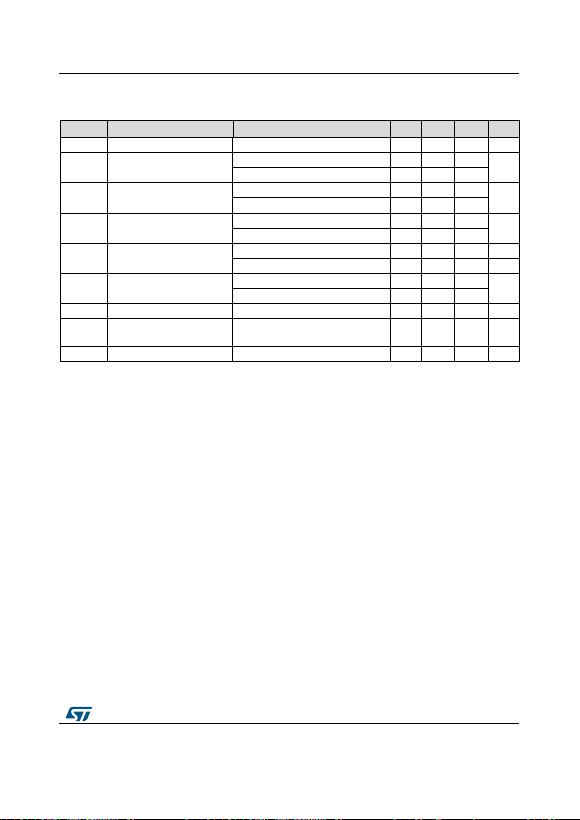L78L
Electrical characteristics
DocID2145 Rev 26
19/45
Refer to the test circuits, TJ = 0 to 125 °C (AC) TJ = -40 to 125 °C (AB), VI =15 V,
IO= 40 mA, CI = 0.33 μF, CO = 0.1 μF unless otherwise specified.
Table 16: Electrical characteristics of L78L09AB and L78L09AC
Symbol
Parameter
Test conditions
Min.
Typ.
Max.
Unit
VO
Output voltage
TJ = 25 °C
8.64
9
9.36
V
VO
Output voltage
IO = 1 to 40 mA, VI = 11.5 to 23 V
8.55
9.45
V
IO = 1 to 70 mA, VI = 15 V
8.55
9.45
∆VO
Line regulation
VI = 11.5 to 23 V, TJ = 25 °C
225
mV
VI = 12 to 23 V, TJ = 25 °C
150
∆VO
IO = 1 to 100 mA, TJ = 25 °C
80
mV
IO = 1 to 40 mA, TJ = 25 °C
40
Id
Quiescent current
TJ = 25 °C
6
mA
TJ = 125 °C
5.5
mA
∆Id
Quiescent current change
IO = 1 to 40 mA
0.1
mA
VI = 12 to 23 V
1.5
eN
Output noise voltage
B = 10 Hz to 100 kHz, TJ = 25 °C
70
µV
SVR
Supply voltage rejection
VI = 12 to 23 V, f = 120 Hz
IO = 40 mA, TJ = 25 °C
37
44
dB
Vd
Dropout voltage
1.7
VElectrical characteristics
L78L
20/45
DocID2145 Rev 26
Refer to the test circuits, TJ = 0 to 125 °C (AC) TJ = -40 to 125 °C (AB), VI= 16 V,
IO = 40mA, CI = 0.33 μF, CO = 0.1 μF unless otherwise specified.
Table 17: Electrical characteristics of L78L10AC
Symbol
Parameter
Test conditions
Min.
Typ.
Max.
Unit
VO
Output voltage
TJ = 25 °C
9.6
10
10.4
V
VO
Output voltage
IO = 1 to 40 mA, VI = 12.5 to 23 V
9.5
10.5
V
IO = 1 to 70 mA, VI = 16 V
9.5
10.5
∆VO
Line regulation
VI = 12.5 to 23 V, TJ = 25 °C
230
mV
VI = 13 to 23 V, TJ = 25 °C
170
∆VO
IO = 1 to 100 mA, TJ = 25 °C
80
mV
IO = 1 to 40 mA, TJ = 25 °C
40
Id
Quiescent current
TJ = 25 °C
6
mA
TJ = 125 °C
5.5
mA
∆Id
Quiescent current change
IO = 1 to 40 mA
0.1
mA
VI = 13 to 23 V
1.5
eN
Output noise voltage
B = 10 Hz to 100 kHz, TJ = 25 °C
60
µV
SVR
Supply voltage rejection
VI = 14 to 23 V, f = 120 Hz
IO = 40 mA, TJ = 25 °C
37
45
dB
Vd
Dropout voltage
1.7
VL78L
Electrical characteristics
DocID2145 Rev 26
21/45
Refer to the test circuits, TJ = 0 to 125 °C (AC) TJ = -40 to 125 °C (AB), VI =19 V,
IO = 40 mA, CI = 0.33 μF, CO = 0.1 μF unless otherwise specified.
Table 18: Electrical characteristics of L78L12AB and L78L12AC
Symbol
Parameter
Test conditions
Min.
Typ.
Max.
Unit
VO
Output voltage
TJ = 25 °C
11.5
12
12.5
V
VO
Output voltage
IO = 1 to 40 mA, VI = 14.5 to 27 V
11.4
12.6
V
IO = 1 to 70 mA, VI = 19 V
11.4
12.6
∆VO
Line regulation
VI = 14.5 to 27 V, TJ = 25 °C
250
mV
VI = 16 to 27 V, TJ = 25 °C
200
∆VO
IO = 1 to 100 mA, TJ = 25 °C
100
mV
IO = 1 to 40 mA, TJ = 25 °C
50
Id
Quiescent current
TJ = 25 °C
6.5
mA
TJ = 125 °C
6
mA
∆Id
Quiescent current change
IO = 1 to 40 mA
0.1
mA
VI = 16 to 27 V
1.5
eN
Output noise voltage
B = 10 Hz to 100 kHz, TJ = 25 °C
80
µV
SVR
Supply voltage rejection
VI = 15 to 25 V, f = 120 Hz
IO = 40 mA, TJ = 25 °C
37
42
dB
Vd
Dropout voltage
1.7
V